# Tower of Hanoi / Rudenko Disk / Rudenko Clips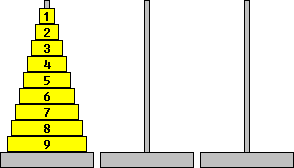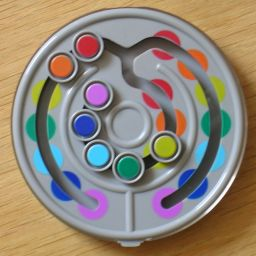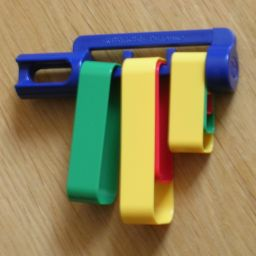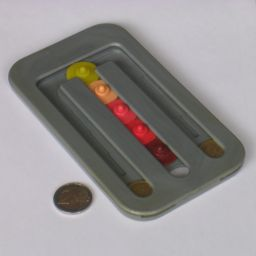This puzzle consists of three pegs, and a stack of circular disks of differing sizes, each of which can be threaded onto a peg. At the start, the disks are all in order on the first peg, from the largest disk at the bottom to the smallest disk at the top. You are only allowed to move one disk at a time from one peg to another, and at no time may a disk be placed on top a smaller disk. The aim of the puzzle is to move all the disks from the first peg to the third. The puzzle can be solved with any number of disks, but it takes longer with more disks.

This puzzle was invented by the French mathematician Edouard Lucas in 1883. The description included with the puzzle when it was originally sold called it a simplified version of a mythical 'Tower of Brahma' in a temple in the Indian city of Benares. This tower consisted of 64 disks, which had to be moved to another position by priests. The legend went that if they completed the task, the tower would crumble and the world would end.Rudenko Matryoshka is a version of the standard Hanoi puzzle where the moving pieces are like traditional nested Matryoshka dolls, with the outer shells being analogous to the smaller disks. The pieces are constrained to move along a track, and there is a locking mechanism is place that is supposed to prevent more than one piece moving at the same time.Rudenko Clips is clever version of the Hanoi puzzle. Its body has a horizontal bar onto which are hung a set of concentric bands. The bar has three sections, and you can move a band from one section to an adjacent one. You can only move the outer band of a section, and can only move it if it is larger than all the bands on the section you move it to. This is slightly more restrictive than the standard Hanoi rules, since you cannot move a band directly between the outer sections but only via the middle section. This means that only the largest band can be moved in one move between the outer sections, while all other bands have to do it in two steps.Rudenko Disk is a disc-shaped version of the puzzle where the moving pieces are beads constrained to move along a track. The pegs are represented by the three connected dead-end tracks. The pieces cannot move further down each track than their starting location. This is slightly less restrictive than the standard Hanoi rules, as it does not restrict the size of the pieces stacked on top of another, only how many can be stacked on it.
Note that the Panex puzzle has a similar rule, but as that puzzle has two towers that need to be exchanged, it is much harder.This unknown, probably Hungarian puzzle uses a mechanism similar to the Rudenko Disk and the Panex puzzle, and like the latter it has three vertical slots for the pegs. It has one tower of five disk-shaped pieces, and the pieces cannot move further down each track than their starting location. The top disk is confined to the horizontal track connecting the pegs. This puzzle has the neat feature that you can insert a coin at the bottom of an outer track, which then acts as a sixth disk. The only way to free the coin is to manoeuvre it to the centre track which has a hole at the bottom. You can even put a coin in both outer tracks for a harder (or more tedious) challenge.

If your browser supports JavaScript, then you can play the Towers of Hanoi by clicking the link below:

## The number of possible positions:

If the disks are placed on the pegs one by one, starting with the largest one, then there are always three choices when placing a disk. Any legal position can be constructed in this manner. There are therefore 3N positions for N disks. At each position (except when all the disks are on one peg) there are 3 possible moves, one which brings you closer to the start, one which brings you closer to the end, and one which brings you closer to having all disks on the middle peg.

The Rudenko disk has more possible positions, but it is very hard to calculate exactly how many.

## Solution to the standard Hanoi puzzle:

The puzzle can be solved by a recursive algorithm. To move a stack of N disks from one peg to another, simply follow the following steps:

1. Move the top N-1 disks of the stack aside, on the spare peg.
2. Move disk N in position on the other peg.
3. Move the stack of N-1 disks on top of disk N.

This method works because this reduces the puzzle of N disks to a puzzle of only N-1 disks. That in turn is reduced to a puzzle of N-2 disks, and so on until the puzzle is reduced to moving a stack containing only 1 disk, which is easy. This algorithm has exactly 2N-1 moves, because from the steps above it is easily seen that Length(N) = 2·Length(N-1) + 1, and Length(1) = 1, and the result then follows by induction. This solution is also minimal, in other words there is no shorter way to solve it, because every solution for N disks where the largest disk moves once must reach the intermediate position with the top N-1 disks on the spare peg. The solution where the largest disk moves more than once is easily seen to be much longer.

The recursive algorithm works very well, but in practice there are several rules of thumb which can guide you:

• Every other move is a move of the smallest disk, i.e. the small disk is moved on move number 1, 3, 5, ... ,2N-1.
• The smallest disk visits each peg in turn, and is never moved back to the peg it came from on its previous move. In fact this is true of all disks (except for the largest), but it is more obvious with the smallest one since it is moved so often.
• On a move not involving the small disk (i.e. moves 2, 4, 6, ...) there is only one possible move choice.
• If the disks are numbered, then an even disk is never placed on top of an even disk, and an odd one never on top of another odd one.
• If there is an even number of disks then the first move takes the small disk to the second peg, with an odd number of disks the first move is to the third peg. This can be seen as an extension of the odd/even rule above; the bases of the pegs are also considered odd or even in such a way that the start and end positions follow the odd/even rule, and then the first move can be deduced.

There are 3N possible positions, and 2N positions are encountered while solving the puzzle directly using the methods above. All the other 3N-2N positions break the odd/even rules by having two disks of the same parity on top of each other (or the largest disk on the middle peg). The odd/even rule always rules out one of the three available moves, so that you can only go forwards to the end or back to the beginning.

If you stop in the middle of solving and forget what your last move was, then you may find yourself reversing previous moves and ending up back at the beginning. The rules of thumb also do not help you when you encounter one of the 3N-2N positions that do not lie on the direct route between the start and the end. In these cases the correct move can be deduced as follows:

1. You want to move the stack of N disks to the third peg. You therefore want to put disk N on the third peg first.
2. If the disk is in position, then the aim is to put all the smaller disks on top of it. If not, then the aim is to put all the smaller disks on the other peg.
3. Repeat the above for this smaller stack of disks.

This is best illustrated by example: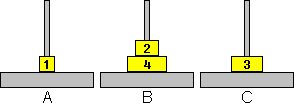Here we want to move disk 4 from peg B to peg C. This means that we need all the smaller disks to be on peg A, so we must move disk 3 from peg C to peg A first. For this we need all disks smaller than 3 to be on peg B. Disk 2 is already on peg B, but disk 1 is not so this has to be moved to peg B. The correct move is therefore disk 1 from A to B.
Similarly if we want to move back to start, to move disk 4 from B to A means that disk 3 and smaller must be on peg C. Disk 3 is already on C, so we must put disk 2 on C next. Disk 1 does not obstruct this, so the correct move is disk 2 from B to C.
If we wanted all the disks on peg B instead, then the correct move would be move disk 1 from A to C. Note that this move does break the odd/even rule (disk 1 moves on top of disk 3), but that is only because the position itself already breaks that rule (disk 2 lies on disk 4).

An interesting pattern emerges if you map out all possible positions in a graph, with lines connecting two positions that differ by a single move. The graph will resemble Sierpinski's gasket; the more disks, the more closely its graph approximates the gasket's fractal triangular shape. The shortest solution is the path along the edge of the triangle, from one corner to the other.

## Solution to the Rudenko Clips:

This puzzle can be solved recursively in a similar way to the standard Hanoi puzzle, except that it has a few more steps. To move a set of N bands from the middle section to the left section, you can follow the following steps:

1. Move the top outer N-1 bands aside, to the right hand side section.
2. Move the Nth band in position to the left section.
3. Move the stack of N-1 bands from the right section to the middle, by reversing the moves from step a.
4. Move the stack of N-1 bands from the middle section to the left.

From these steps it is easy to see that Length(N) = 3·Length(N-1) + 1 and Length(1) = 1. It can then be proved by induction that this algorithm takes exactly (3N-1)/2 moves. This solution is also optimal.

If you consider the movement of the outer band from one side to the other to be a single move instead of two, then the solution obviously takes fewer moves. The moves involving the N-1 smaller bands are like the solution above and take (3N-1-1)/2 moves. These moves alternate with single moves of the outer band, which must therefore be moved 1 + (3N-1-1)/2 times. The total number of moves under this definition is then 3N-1.

Rudenko Clips has 7 moving pieces, so the number of moves needed to move all of them to or from the middle section is (37-1)/2 = 1093, or 36 = 729 if the large band may switch sides in a single move.

At any point in the middle of the solution, there are only two moves possible - moving the large band from one side to the other, or moving the second largest accessible piece. You can deduce what the next move is by looking at the smallest band that is not yet solved, determining how it needs to be moved, and deducing what conditions this imposes on the next larger band. Then use the same reasoning on that band, and so on until you get to a band that can be moved.

We can map out the positions in a graph in the same way as before. Instead of a Sierpinski's gasket, we now get a single line going through all 3N positions. In every triangle in the original graph, (the middle of) one of the three sides represents a long move between the outer pegs. These have been removed leaving only a single fractal-like path.

## Solution to the Hanoi Coin Puzzle:

The solution to this puzzle is the same as the Rudenko Clips above. The top piece blocks any other piece from moving directly between the outer tracks, and so the Rudenko Clips solution works for this puzzle too. This puzzle is also slightly different, because there is no physical obstruction to placing a 'large' disk on top of a 'smaller' one. It turns out however that if you do so, then there will not be enough room left to solve the puzzle, and you reach a dead end. Therefore always only do moves that leave the moving disk at the same height as before.

There are 5 moving pieces, so the number of moves needed to move all of them to or from the middle section is (35-1)/2 = 121, or 34 = 81 if the top piece may switch sides in a single move.

It is hard to determine how many reachable positions this puzzle has if you include all the dead-end positions where larger disks are placed on top of smaller ones. I used a computer program to enumerate them, and the results are shown below.

DisksRegular positionsReachable positions
1 3 3
2 9 9
3 27 27
4 81 99
5 243 423
6 729 2,007
7 2,187 10,167
8 6,561 53,739
9 19,683 292,299
10 59,0491,622,079
11177,1479,133,371

## Solution to the Rudenko Disk:

The standard Hanoi solution can be applied to this puzzle. It has 7 moving pieces, so it takes 27-1 = 127 moves if solved in this way. It is however possible to take advantage of the fact that it is less restricted than the normal Hanoi puzzle. For example, a four disk puzzle can be solved in 13 moves instead of 15 as follows: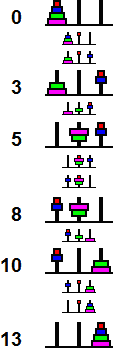The shortcut above generalises, and allows you to reduce the problem of moving a stack of N disks to the simpler problems of moving a stack of N-2 disks three times, and moving the two largest disks twice. This leads to a solution for which the length satisfies the equality Length(N) = 3·Length(N-2) + 4. From this and the fact that Length(1) = 1, and Length(2) = 3, we can prove by induction that if N is even, say N=2n, then Length(2n) = 5·3n-1 - 2, and if N is odd, N=2n-1, then Length(2n-1) = 3n - 2.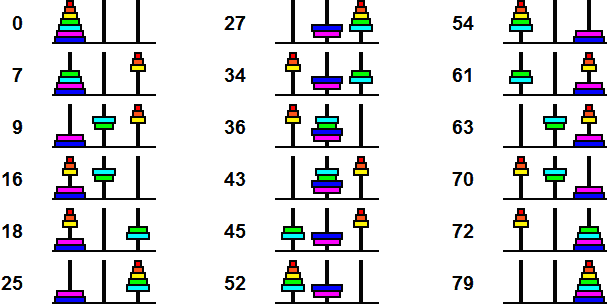I believe that it is impossible to do better than the above solution whatever the size of the puzzle. I have verified this by computer up to N=10 disks, but not proven it mathematically. The following table shows the results for various sizes of this puzzle.

Standard HanoiRudenko Disk
Number
of disks
Solution
Length
Number of
positions
Optimal
Solution Length
Number of
Optimal Solutions
Number of
positions
DiameterAntipodes
1 1 3 1 1 3 12 3 9 3 1 9 3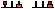3 7 27 7 2 33 8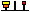4 15 81 13 1 141 17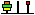5 31 243 25 8 693 33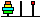6 63 729 43 1 3813 59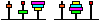7 127 2187 79 512 22797107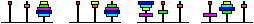8 255 6561133 1 144213187
9 51119683241134217728 947853335
10102359049403 16396837570